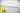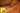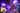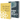# Linear Regression with Gradient Descent in JavaScript

by Robin WieruchRecently I started to take the Machine Learning course by Andrew Ng on Coursera. So far, it is a blast and I am so keen to apply all my learnings in math from university. Starting right into web development after university, I never had the opportunity to apply those learnings when implementing web applications. Now, it is refreshing to see use cases in machine learning where those learnings could be used.

In the following article, I want to guide you through building a linear regression with gradient descent algorithm in JavaScript. Since JavaScript is the programming language that I feel most comfortable with, I try to apply my learnings in machine learning in JavaScript as long as I can. Afterward, I hope to find the time to transition these learnings to Python. The article doesn't give you an in depth explanation of linear regression and gradient descent, so if you are interested in those topics, I highly recommend the referenced machine learning course. Furthermore, I am just learning it myself, so if there are any mistakes on the way, please help me out.

## Solving a Problem with Machine Learning

By using machine learning (ML), we want to solve a real problem. A common problem to solve when learning ML is predicting housing prices in Portland. If you think about the problem, what would be the characteristics of a house in an urban area to predict its price? The size? The year when it was built? The distance to the city centre? The point is that there are endless of characteristics for a house that could contribute to the price of a house. These characteristics of one date (house) in a data set (houses) are called features. For instance, the housing price and the size in square meter are features.

In order to simplify the process of learning ML in the article by using linear regression and gradient descent, let's assume that the only feature that affects a housing price in Portland is its size in square meters. That way, we can apply an univariate linear regression that simplifies the algorith, because we are only using one feature. After understanding the basics, you can scale it up to a multivariate linear regression when using multiple features for a house.

Imagine you would need to plot a data set of those houses in a chart whereas the features price and size are in relation to each other.

`Price +      |      |      |                    0              0      |                               0      |      |      |      |     0      |      |                0      |      |      |      +---------------------------------------+                                           Size`

You can draw a line through the data set to approximate the prices of other houses that are not within the data set. The line can be called best-fit prediction line. Linear regression establishes a relationship between dependent variable (e.g. price), also called output or label, and one or more independent variables (e.g. size of a house) using a best fit straight line or regression line.

`Price +      |                                 XXX      |                              XXX      |                    0      XXX     0      |                        XXX    0      |                     XXX      |                  XXX      |               XXX      |     0      XXX      |         XXX      |      XXX       0      |   XXX      |XXX      |      +---------------------------------------+                                           Size`

Now the line could be used to predict the price of a house that is new on the housing market. The bigger the data set, in Machine learning terms it is called the training set, the more accurate the line that cuts through it and the more accurate the prediction for a new house.

`Price +      |                                 XXX      |                              XXX      |                    0      XXX     0      |                        XXX    0      |                     XXX      |------------------0XX      |               XXX|      |     0      XXX   |      |         XXX      |      |      XXX       0 |      |   XXX            |      |XXX               |      |                  |      +---------------------------------------+                                           Size`

In this simplified exampled, the prediction is only estimated by one feature, the price, but in a real problem solving use case it could be a larger amount of features in order to have a realistic prediction.

In addition, in the example, I have chosen a linear model, so a straight line, to estimate the housing prices. But you could choose to apply a different model type to fit your training set. It could be a exponential model or cubic model with a curved line instead. In the end, you can decide on the best fitting model depending on the cost returned from the cost function.

## Hypothesis and Cost Function in JavaScript

In order to predict a price of a house with a particular size, we need to come up with the straight line that goes through the data set as most predicting as possible. The straight line can be defined as a function. The function is called hypothesis in machine learning. The function can be defined as `h(x) => thetaZero + thetaOne * x` for a univariate linear regression problem whereas the chosen model type is a linear model. Otherwise it would be a . In JavaScript, it can be expressed as:

`const hypothesis = x => thetaZero + thetaOne * x;`

If you remember from your calculus classes in math, in this case the thetaOne of the function defines the slope and the thetaZero the intersection on the y-axis. In the end, it is a straight line in your 2-dimensional coordinate system.

The end goal would be the perfect hypothesis to predict the price for a new house. But we cannot simply guess the hypothesis. So how can we know about the two parameters thetaZero and thetaOne in the hypothesis function? The cost function in machine learning is used to calculate how well the hypothesis function is performing regarding the data set. The cost function calculates the sum of the squared differences between actual and predicted outcome. Note that it is the square, because the difference can be negative.

`Price +      |                                 XXX      |                              XXX  |      |                    0      XXX |   0      |                    |   XXX    0      |                    |XXX      |                  XXX      |               XXX      |     0      XXX |      |     |   XXX    |      |     |XXX       0      |   XXX      |XXX      |      +---------------------------------------+                                           Size`

In JavaScript, the cost function can be defined as the following function. The x represents an array of housing sizes (e. g. [40, 77, 110]), y an array of housing prices (e.g. [78, 130, 190]) and M the size of the training set (e.g. 3):

`const cost = () => {  let sum = 0;   for (let i = 0; i < M; i++) {    sum += Math.pow(hypothesis(x[i]) - y[i], 2);  }   return sum / (2 * M);}`

Depending on the parameters of the hypothesis function, the cost function has a different outcome. For instance, in the next example the cost is higher than the cost of the previous example. The parameters of the hypothesis function are not performing well.

`Price +      |      |      |                    0              0      |                    |          0   |      |                    |          |   |      |                    |          |   |      |                    |          |   |      |     0              |          |   |      |     |              |          |   |XXXX      |     |          0   |      XXXXXXXXX      |     |          | XXXXXXXXX      |     |   XXXXXXXXX      |XXXXXXXXX      +---------------------------------------+                                           Size`

The ideal outcome would be a minimum of costs. But that's not going to happen and could .

`Price +      |                                 XX0      |                              X0X      |                           XXX      |                        XXX      |                     XXX      |                  XX0      |               X0X      |            XXX      |         XXX      |      XXX      |   XX0      |XXX      |      +---------------------------------------+                                           Size`

The best we can do is finding the minimum costs for the two parameters thetaZero and thetaOne in the hypothesis function. So how to calculate the best fitting parameters, thetaZero and thetaOne, for the hypothesis function? Can we make use of the cost function in our tool set now?

By going through many values of thetaZero and thetaOne, we could find a best-fit linear model that minimizes the cost function eventually. Apart from going through all the possible values and variations for thetaZero and thetaOne manually, is there a better way to define thetaZero and thetaOne to minimize the cost? It happens that there is a way: gradient descent.Imagine a hilly landscape and you are standing on one of the hills. This one position is described by one pair of parameters thetaZero and thetaOne. The height to the sea level describes your cost. The goal is to reduce the cost. So what would be the best approach to reduce the cost in the metaphor? You have to find the vale in the hilly landscape! In math it is called the global minima (or the local minima in case of multiple minimas in a multivariate linear regression). So what is a gradient descent algorithm doing in simple words?

1. find the steepest downward step you can perform
2. take the step of size alpha in that direction (alpha = learning rate)
3. repeat until you converge to a local minima (learning)

If the learning rate alpha is too high, it can be that you just pass through the local minima and end up on another hill. So the learning rate should be low to converge slowly to the local minima but not too low because otherwise it could take ages.

`const LEARNING_RATE = 0.0003;`

Your position in the hilly landscape can be chosen by random in the beginning. It can happen that it is already a vale (local minima) and not a hill. That would be a lucky shot. Often it starts for thetaZero = 0, thetaOne = 0 which concludes to `h(x) => 0x + 0`. So you start with a random hypothesis, but try to adjust its paramaters to minimize the cost by using gradient descent.

`let thetaOne = 0;let thetaZero = 0;`

There is one interesting catch to the story when having a multivariate linear regression which is not discussed in this article (multiple features instead of one feature). It doesn't matter for the sake of explaining an univariate linear regression by using gradient descent, but I found it an interesting addition to the metaphor. In the beginning, you decided randomly to stay on one of the hills in the hilly landscape. By using gradient descent, you went down the hill to your local vale. The local vale can be described as one local minima. When using multiple features instead of only one, it can happen that you have multiple local minima and one global minima. Depending on the randomly selected hill in the hilly landscape in the beginning, you might end up in different local vales that doesn't need to be necessary the global minima.

In JavaScript, a gradient descent algorithm for a univariate linear regression could be expressed in one function that needs to be executed until the results for thetaZero and thetaOne converge. Arriving at this function requires you the hypothesis function, the cost function and calculus for computing the partial derivative of the cost function. This article will not go into detail about these steps, but I recommend again to take the Machine Learning course.

`const learn = (alpha) => {  let thetaZeroSum = 0;  let thetaOneSum = 0;   for (let i = 0; i < M; i++) {    thetaZeroSum += hypothesis(x[i]) - y[i];    thetaOneSum += (hypothesis(x[i]) - y[i]) * x[i];  }   thetaZero = thetaZero - (alpha / M) * thetaZeroSum;  thetaOne = thetaOne - (alpha / M) * thetaOneSum;}`

By running the learn function many times until the result of thetaZero and thetaOne converge, the cost is minimzed and we will get as result our fitting hypothesis function to draw the straight line through our data set. By having the hypothesis function, you can predict new housing prices based on the size of square meter of a house.

You can find an example of the linear regression with gradient descent in JavaScript in one of my GitHub repositories. If you like it, make sure to star it. It displays the result as well, even though React is used to do it in an efficient way. I didn't use any local state management of React to store the machine learning variables, because I wanted to keep the machine learning layer separated from the view layer as much as possible for demonstrating linear regression with gradient descent in JavaScript.

In conclusion, I hope the walkthrough in JavaScript was useful for you to understand linear regression and gradient descent. If you are sharing the article, it would make me aware of people actually wanting to read more about those topics, so I can write more about them in the future. The next article in this series shows a . I learn the topic myself, so please leave a comment if there is any mistake.## Linear Algebra in JavaScript with Matrix Operations

When I recently started to dive into the topic of machine learning, I had to relearn all the things I have studied about linear algebra, stochastic and calculus at school and university. I took a…## Neural Networks in JavaScript with deeplearn.js

A couple of my recent articles gave an introduction into a subfield of artificial intelligence by implementing foundational machine learning algorithms in JavaScript (e.g. linear regression with…